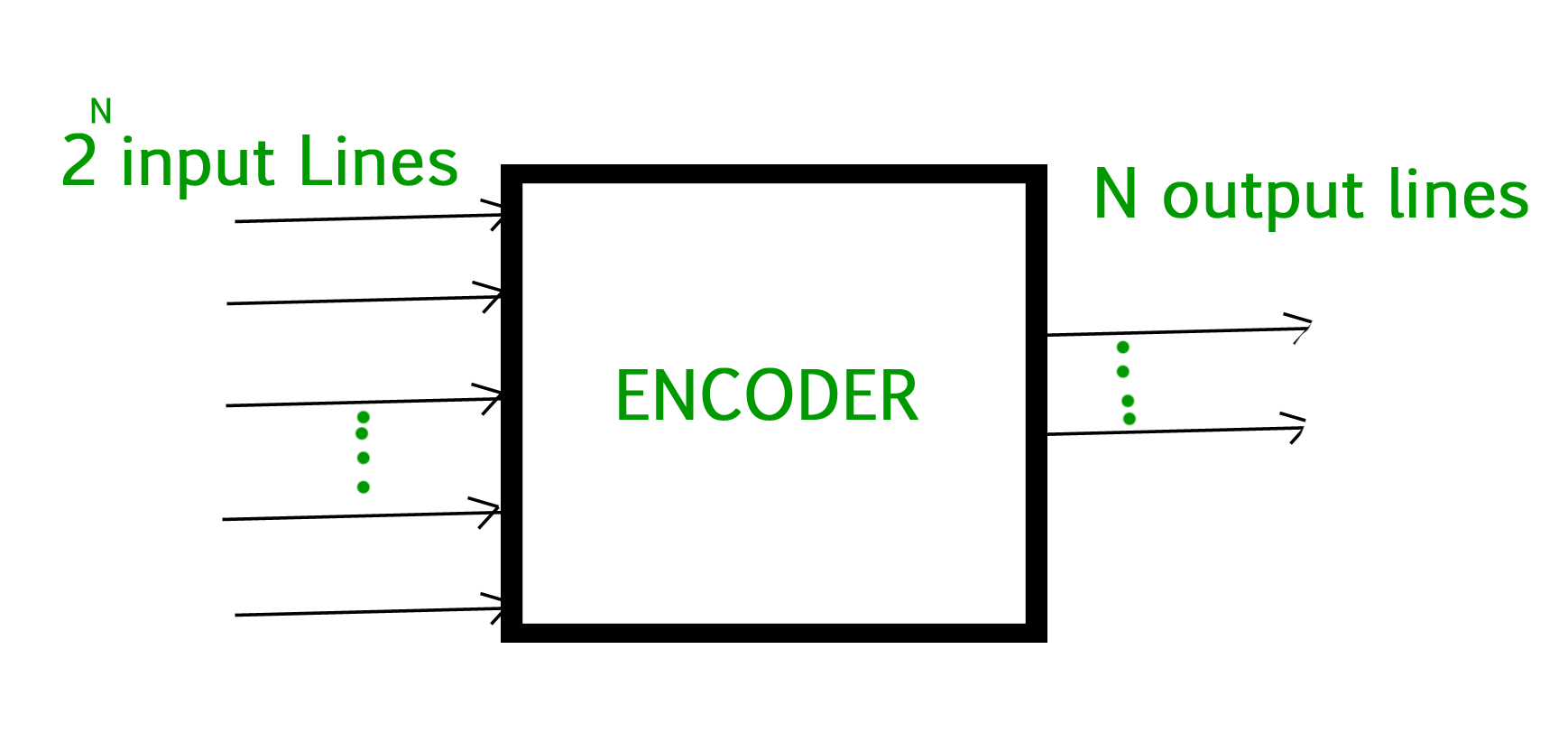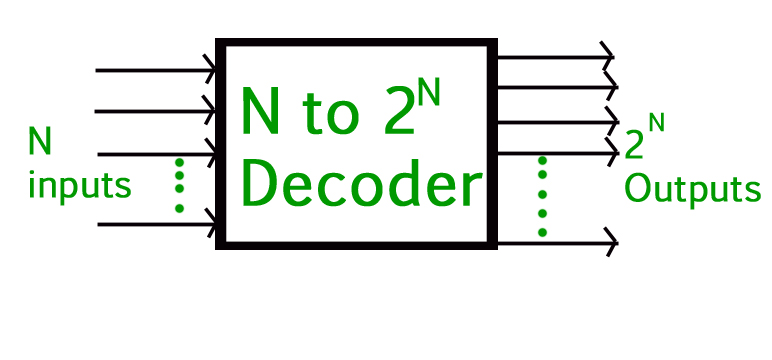Related Articles

# Difference between Encoder and Decoder

• Difficulty Level : Medium
• Last Updated : 13 Oct, 2020

Combinational logic is the concept in which two or more input state define one or more output state. Encoder and Decoder are the combinational logic circuits. In which we implement combinational logic with the help of boolean algebra.

To encode something is to convert an unambiguous piece of information into a form of code that is not so clearly understood and the device which performs this operation is termed ad Encoder.

1. Encoder :
An Encoder is a device that converts the active data signal into a coded message format or it is a device that coverts analogue signal to digital signals. It is a combinational circuit, that converts binary information in the form of a 2N input lines into N output lines which represent N bit code for the input. When an input signal is applied to an encoder then logic circuitry involved within it converts that particular input into coded binary output.To decode is to perform the reverse operation :converting a code back into an unambiguous form code and the device which perform this operation is termed as Decoder.

2. Decoder :
A decoder is also a combinational circuit as encoder but its operation is exactly reverse as that of the encoder. A decoder is a device that generates the original signal as output from the coded input signal and coverts n lines of input into 2n lines of output. An AND gate can be used as the basic decoding element because it produces a high output only when all inputs are high.Difference between encoder and decoder :

S.No.ENCODERDECODER
1Encoder circuit basically converts the applied information signal into a coded digital bit stream.Decoder performs reverse operation and recovers the original information signal from the coded bits.
2In case of encoder, the applied signal is the active signal input.Decoder accepts coded binary data as its input.
3The number of inputs accepted by an encoder is 2n.The number of input accepted by decoder is only n inputs.
4The output lines for an encoder is n.The output lines of an decoder is 2n.
5The encoder generates coded data bits as its output.The decoder generates an active output signal in response to the coded data bits.
6The operation performed is simple.The operation performed is complex.
7The encoder circuit is installed at the transmitting end.The decoder circuit is installed at the receiving side.
8OR gate is the basic logic element used in it.AND gate along with NOT gate is the basic logic element used in it.
9It is used in E-mail, video encoders etc.It is used in Microprocessors, memory chips etc.

Attention reader! Don’t stop learning now.  Practice GATE exam well before the actual exam with the subject-wise and overall quizzes available in GATE Test Series Course.

Learn all GATE CS concepts with Free Live Classes on our youtube channel.

My Personal Notes arrow_drop_up